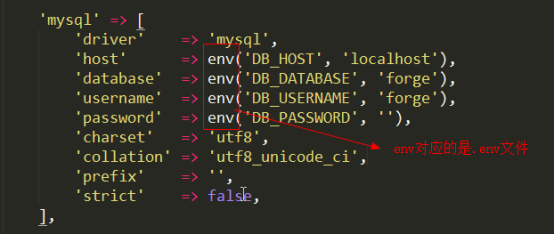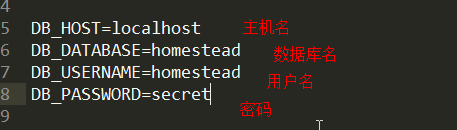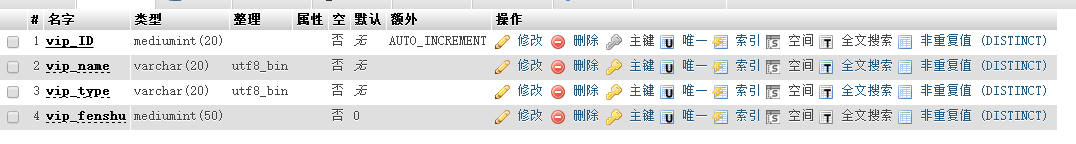Laravel数据库操作的三种方式在app->Http->Controllers目录下新建一个Student控制器，StudentController.php。 StudentController.php代码如下：

<?php
namespace App\Http\Controllers;
class StudentController extends Controller {

}
1.查询操作

在Student控制器里添加一个test1方法，查询用的是DB类的静态方法select()，参数是原生的sql语句，返回的是一个二维数组。dd()是laravel提供的方法，可以将一个数组以节点树的形式展示出来。具体代码如下：

public function test1()
{
$student=DB::select("select * from vipinfo"); //返回一个二维数组$student
var_dump($student); //以节点树的形式输出结果 dd($student);
}

路由配置： Route::get('test1',['uses'=>'StudentController@test1']);

URL访问：http://localhost/laravel/public/index.php/test1 ，则会打印出结果。

2.新增操作

$bool=DB::insert("insert into vipinfo(vip_ID,vip_name,vip_type,vip_fenshu) values(?,?,?,?)",[5,'小明','出行',670]); var_dump($bool);
//新增成功则返回true。
3. 更新操作

$bool=DB::update('update vipinfo set vip_fenshu= ? where vip_ID= ? ',[700,5]); var_dump($bool);  //更新成功返回true
4. 删除操作

$num=DB::delete('delete from vipinfo where vip_ID= ?',); echo$num;

laravel查询构造器提供了方便流畅的接口，用来建立及执行数据库查找语法。使用了pdo参数绑定，使应用程序免于sql注入，因此传入的参数不需要额外转义特殊字符。基本上可以满足所有的数据库操作，而且在所有支持的数据库系统上都可以执行。

1.使用查询构造器实现增删改查

1）新增

$bool=DB::table("vipinfo")->insert(['vip_ID'=>6,'vip_name'=>'zls','vip_type'=>"出行",'vip_fenshu'=>800]); echo$bool;  //返回bool值
//如果想得到新增的id，则使用insertGetId方法
$id=DB::table("vipinfo")->insertGetId(['vip_ID'=>5,'vip_name'=>'wyp','vip_type'=>"出行",'vip_fenshu'=>800]); echo$id;
//插入多条数据
$bool=DB::table("vipinfo")->insert([ ['vip_ID'=>5,'vip_name'=>'wyp','vip_type'=>"出行",'vip_fenshu'=>800], ['vip_ID'=>6,'vip_name'=>'zls','vip_type'=>"出行",'vip_fenshu'=>800], ]); echo$bool;  //返回bool值

（2）修改

$bool=DB::table("vipinfo")->where('vip_ID',6)->update(['vip_fenshu'=>500]); echo$bool;
//自增
$bool=DB::table("vipinfo")->where('vip_ID',6)->increment("vip_fenshu");//自增1$bool=DB::table("vipinfo")->where('vip_ID',6)->increment("vip_fenshu",3);//自增3
echo $bool; //自减$bool=DB::table("vipinfo")->where('vip_ID',6)->decrement("vip_fenshu");//自1
$bool=DB::table("vipinfo")->where('vip_ID',6)->decrement("vip_fenshu",3);//自增3 echo$bool;
//自增时再修改其他字段
$bool=DB::table("vipinfo")->where('vip_ID',6)->increment("vip_fenshu",3,['vip_name'=>'dbdibi']);//自增3 （3）删除 $num=DB::table("vipinfo")->where('vip_ID',6)->delete();//删除1条
$num=DB::table("vipinfo")->where('vip_ID','>',4)->delete();//删除多条 echo$num;  //删除的行数
$num=DB::table("vipinfo")->truncate();//删除整表，不能恢复，谨慎使用 （4）查询 //get()返回多条数据$student=DB::table("vipinfo")->get();
var_dump($student); //first()返回1条数据$student=DB::table("vipinfo")->first();  //结果集第一条记录
$student=DB::table("vipinfo")->orderBy('vip_ID','desc')->first();//按vip_ID倒序排序 var_dump($student);
//where()条件查询
$student=DB::table("vipinfo")->where('vip_ID','>=',2)->get(); //一个条件$student=DB::table("vipinfo")->whereRaw('vip_ID> ? and vip_fenshu >= ?',[2,300])->get(); //多个条件
dd($student); //pluck()指定字段,后面不加get$student=DB::table("vipinfo")->pluck('vip_name');
dd($student); //lists()指定字段，可以指定某个字段作为下标$student=DB::table("vipinfo")->lists('vip_name','vip_ID');   //指定vip_ID为下标
dd($student);$student=DB::table("vipinfo")->lists('vip_name');   //不指定下标，默认下标从0开始
//select()指定某个字段
$student=DB::table("vipinfo")->select('vip_name','vip_ID')->get(); dd($student);
//chunk()每次查n条
$student=DB::table("vipinfo")->chunk(2,function($students){  //每次查2条
var_dump($students); if(.......) return false; //在满足某个条件下使用return就不会再往下查了 }); 2.使用聚合函数 //count()统计记录条数$nums=DB::table("vipinfo")->count();
echo $nums; //max()某个字段的最大值,同理min是最小值$max=DB::table("vipinfo")->max("vip_fenshu");
echo $max; //avg()某个字段的平均值$avg=DB::table("vipinfo")->avg("vip_fenshu");
echo $avg; //sum()某个字段的和$sum=DB::table("vipinfo")->sum("vip_fenshu");
echo $sum; 四、数据库操作之 - Eloquent ORM 1.简介、模型的建立及查询数据 简介：laravel所自带的Eloquent ORM 是一个ActiveRecord实现，用于数据库操作。每个数据表都有一个与之对应的模型，用于数据表交互。 建立模型，在app目录下建立一个Student模型，即Student.php，不需要带任何后缀。 <?php namespace App; use Illuminate\Database\Eloquent\Model; class Student extends Model{ //指定表名 protected$table= 'vipinfo';
//指定主键
protected $primaryKey= 'vip_ID'; }  在Student控制器里增加一个test3方法，配置路由Route::get('test3',['uses'=>'StudentController@test3']); public function test3(){ // all()方法查询所有数据$studnets=Student::all();
dd($studnets); //find()查询一条，依据主键查询。findOrFail()查找不存在的记录时会抛出异常$student=Student::find(5);  //主键为5的记录
var_dump($student['attributes']); //查询构造器的使用,省略了指定表名$student=Student::get();
var_dump($student); } 2 . 新增数据、自定义时间戳、批量赋值 （1）使用save方法新增 laravel会默认维护created_at,updated_at 两个字段，这两个字段都是存储时间戳，整型11位的，因此使用时需要在数据库添加这两个字段。如果不需要这个功能，只需要在模型里加一个属性：public$timestamps=false; 以及一个方法，可以将当前时间戳存到数据库

protected function getDateFormat(){
return time();
}

$student=new Student(); //设定数据$student->vip_name='xiaoming';
$student->vip_type='出行';$student->vip_fenshu=900;
$bool=$student->save();  //保存
echo $bool; 从数据库里取得某条记录的时间戳时，默认取得的是按日期格式化好的时间戳，如果想取得原本的时间戳，则在模型里增加asDateTime方法。 protected function asDateTime($val){
return $val; } （2）使用create方法新增时，需要在模型里增加： protected$fillable=['vip_name','vip_fenshu','vip_type'];   //允许批量赋值的字段

控制器里写：

Student::create(['vip_name'=>'mmm','vip_fenshu'=>999,'vip_type'=>'出行']);

3）firstOrCreate()以属性查找记录，若没有则新增

$student=Student::firstOrCreate(['vip_name'=>'mmm']); echo$student;

4）firstOrNew()以属性查找记录，若没有则会创建新的实例。若需要保存，则自己调用save方法()

$student=Student::firstOrNew(['vip_name'=>'mmm']);$student->save();
echo $student; 3. 修改数据 //通过模型更新数据$student=Student::find(2);
$student->vip_fenshu=10000;$student->save(); //返回bool值
//通过查询构造器更新
$num=Student::where('vip_ID','>',2)->update(['vip_fenshu'=>2000]); echo$num;  //返回更新的行数

4.  删除数据

//(1)通过模型删除数据
$student=Student::find(11);$student->delete(); //返回bool值
//(2)通过主键删除
$num=Student::destroy(10); //删除主键为10的一条记录 echo$num; //返回删除的行数
$num=Student::destroy(10,5); //删除多条 或者$num=Student::destroy([10,5]);
echo \$num; //返回删除的行数